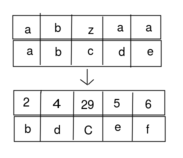String Sets
Tag(s):

## Combinatorics, Hard, Inclusion-Exclusion, Mathematics, generating functions

Problem
Editorial
Analytics

Let's define the weight of an English letter (lowercase or uppercase) in the following way: a has weight 1, b = 2, and so on until z = 26; A = 27 and so on until Z = 52. The weight of an alphabetic string is the sum of weights of all letters it contains, modulo M.

Next, let's define the sum of two strings X and Y of length N consisting of lowercase English letters as a string Z of length N such that for each valid i, the weight of the i-th character of Z is the sum of weights of the i-th characters of X and Y. For example, the sum of strings "abzaa" and "abcde" is the string "bdCef".You are given a string S of lowercase English letters with length N and two integers M and K. You need to select a string B (also consisting only of lowercase English letters) such that the sum of strings S and B has weight K.

Can you find the number of ways to select the string B modulo $10^9+7$?

Constraints

• $1 \le N \le 200,000$

• $100,000 \le M \le 1,000,000,007$

• $0 \le K < M$

• the string S will consist only of lowercase English letters

Input format

The first line of the input contains three space-separated integers N, M and K.

The second line contains a single string S of length N.

Output format

Print a single line containing one integer — the answer modulo $10^9+7$.

SAMPLE INPUT
7 21 13
abcacba
SAMPLE OUTPUT
382471566
Explanation

One possible string B is "abucdef". The sum of "abcacba" and "abucdef" is "bdxdggg". The weight of this string is $2+4+24+4+7+7+7 = 55 \equiv 13$ modulo $21$.

Note that $M = 21$ in the sample. This is strictly for explanatory purposes; the constraint $10^5 \le M \le 10^9+7$ will hold in all real tests.

Time Limit: 3.0 sec(s) for each input file.
Memory Limit: 256 MB
Source Limit: 1024 KB
Marking Scheme: Marks are awarded when all the testcases pass.
Allowed Languages: Bash, C, C++, C++14, Clojure, C#, D, Erlang, F#, Go, Groovy, Haskell, Java, Java 8, JavaScript(Rhino), JavaScript(Node.js), Julia, Kotlin, Lisp, Lisp (SBCL), Lua, Objective-C, OCaml, Octave, Pascal, Perl, PHP, Python, Python 3, R(RScript), Racket, Ruby, Rust, Scala, Swift, Swift-4.1, TypeScript, Visual Basic

## CODE EDITOR

Initializing Code Editor...

## This Problem was Asked inChallenge Name

December Circuits '17

OTHER PROBLEMS OF THIS CHALLENGE
• Data Structures > Advanced Data Structures
• Algorithms > Graphs
• Algorithms > Searching
• Data Structures > Arrays
• Algorithms > Dynamic Programming